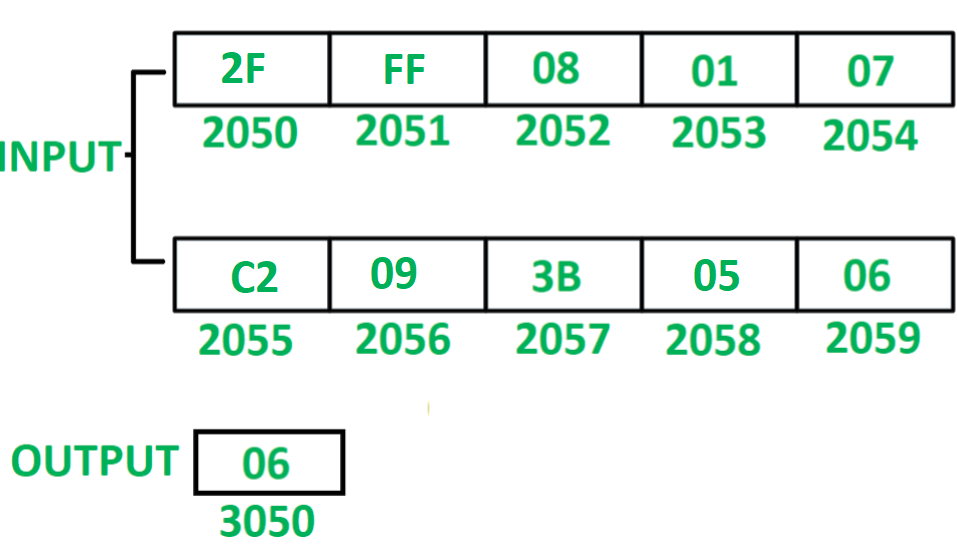# 8085 program to count number of elements which are less than 0A

• Last Updated : 20 Jun, 2018

Problem – Write an assembly language program in 8085 microprocessor to count number of elements which are less than 0A in series of 10 numbers.

Example –Assumption – Series of 10 numbers is stored from starting memory location 2050. Value of count is stored at memory location 3050

Algorithm –

1. Initialize register H with 20 and register L with 4F so that indirect memory points to memory location 204F.
2. Initialize register C with 00 and register D with 0A.
3. Increment indirect memory by 01.
4. Move the content of M in accumulator A.
5. Compare the content of A with 0A by help of CPI instruction. This instruction will update flags of 8085.
6. Check if carry flag is set, if true then increment content of C by 01.
7. Decremented content of D by 01.
8. Check if zero flag is reset, if true then jump to step 3.
9. Move the content of C to A.
10. Store the content of A to memory location 3050.

Program –

2000 LXI H 204F H <- 20, L <- 4F
2003 MVI C, 00 C <- 00
2005 MVI D, 0A D <- 0A
2007 INX H M <- M + 01
2008 MOV A, M A <- M
2009 CPI 0A A – 0A
200B JNC 200F Jump if CY = 0
200E INR C C <- C + 01
200F DCR D D <- D – 01
2010 JNZ 2007 Jump if ZF = 0
2013 MOV A, C A <- C
2014 STA 3050 M <- A
2017 HLT END

Explanation – Registers A, C, D, H, L are used for general purpose.

1. LXI H 204F: assign 20 to register H and 4F to register L
2. MVI C, 00: assign 00 to register C
3. MVI D, 0A: assign 0A to register D
4. INX H: increment indirect memory location by 01
5. MOV A, M: moves the content of indirect memory location M to accumulator A
6. CPI 0A: subtracts 0A from content of A and update the flags of 8085
7. JNC 200F: jump to memory location 200F if CY = 0
8. INR C: increment content of C by 01
9. DCR D: decrement content of D by 01
10. JNZ 2007: jump to memory location 2007 if ZF = 0
11. MOV A, C: moves the content of C to A
12. STA 3050: store the content of A in memory location 3050
13. HLT: stops executing the program and halts any further execution
My Personal Notes arrow_drop_up
Recommended Articles
Page :